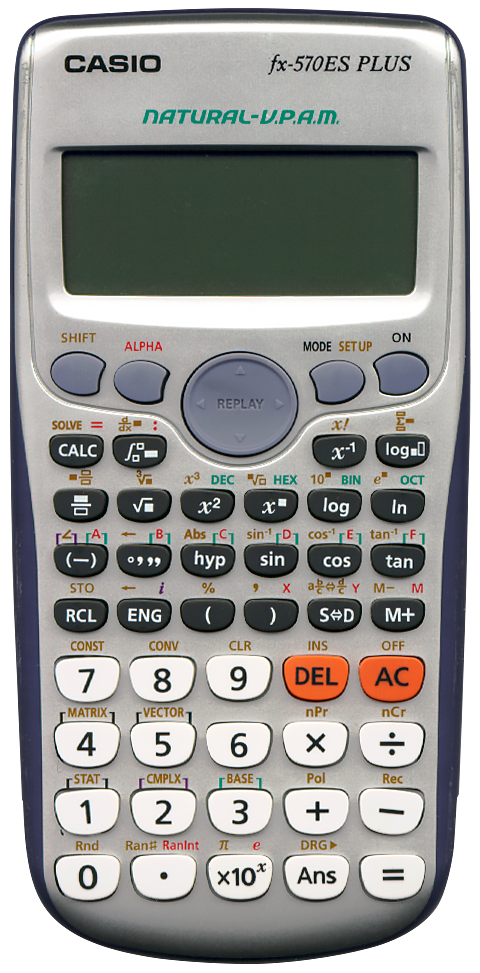# CASIO fx‑570ES PLUS

## Description

 Brand: CASIO Model: fx-570ES PLUS Type: Scientific calculator Picture:Batteries: AAA x 1 Lifetime: Introduced: unknown Terminated: unknown Notes: A 10+2 digit scientific calculator for school and study purposes with "Natural textbook display". In the calculator's "SETUP" one can specify its output format. One can choose between "MathO" and "LineO". When set to the former, the result of "SIN(π/4" (yes you may omit the closing bracket!) will be √2/2. When using the latter format the result will of course be "0.7071067812". The calculator should be set to radians of course. A very capable calculator. Does integration, differentiation, solves equations, does BASE-N calculations, knows complex numbers, matrix- and vector math, and has a library of mathematical constants and conversions (for these one needs a table from the manual because they have to be addressed by index number). All in all, working on this calculator feels very natural. The team that has designed it knows what the user would expect. For instance, when one has a number displayed and one presses '√▖' and '=', the calculator knows one wants to calculate "√(Ans". Earlier calculators had S-V.P.A.M. (Super Visually Perfect Algebraic Method). This calculator extends that to NATURAL-V.P.A.M.